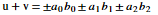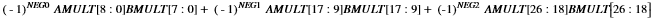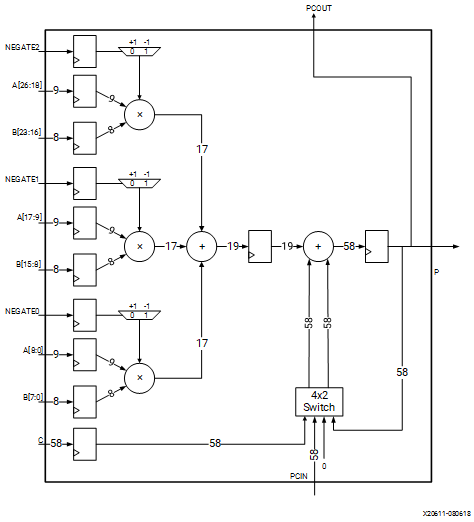# Dot Product Unit

## Versal ACAP DSP Engine Architecture Manual (AM004)

Document ID
AM004
Release Date
2022-09-11
Revision
1.2.1 English

The INT8 mode is used to implement the dot product unit (see the following figure for a simplified block diagram), optimized for neural network and machine learning applications. It computes the inner product of two vectors a = (a0, a1, a2) and b = (b0, b1, b2) where ai is a 9-bit two’s complement fixed point number and bi is an 8-bit two’s complement number. The dot product also supports element-wise product negation with the pins NEGATE[2:0]. This unit computes the partial results u and v such that:Taking pipelining into account, the dot product unit computes the following:Where NEG0, NEG1, and NEG2 are registered versions of NEGATE[2:0] or the signals themselves. AMULT and BMULT are the vector 9 × 8 multiplier inputs. See Figure 1. Similar to the scalar fixed-point ALU, the negation pins do not cause internal overflow, even if any of the inputs is the most negative two’s complement number (all zeros except for the sign bit). Because the dot-product unit is derived from the scalar ALU, other than the pre-adder and the 27 x 24 multiplier, all other features function identically.

Figure 1. Simplified Two’s Complement Dot Product Unit (INT8 mode)Note: All input signals can bypass their input registers.